# Trying to understand space-time diagrams

• I
• Kashmir
A scalar product is a product of vectors that produces a scalar. It is not an inner product, which necessarily comes with the requirement of positive definiteness.f
IMHO there is an element of overthinking in this thread. Especially for a first exposure to spacetime diagrams.

•apostolosdt and vanhees71
IMHO there is an element of overthinking in this thread. Especially for a first exposure to spacetime diagrams.
Maybe the level of the thread should go to "B".

These diagrams may help you appreciate that "rapidity" can be thought of as an "angle",
when used properly. As with any tool, one must learn how to use it and how not-touse it.

Note the use of an arc of the "Minkowski circle"...
so we don't fall into the trap of thinking in terms of Euclidean geometry, as @vanhees71 warns us about.

View attachment 314576

View attachment 314567
View attachment 314568

From
https://physics.stackexchange.com/questions/667907/lorentz-transform-moving-length-to-moving-length
View attachment 314569View attachment 314570

In my opinion, since we teach students to use trigonometry to solve free-body diagrams,
we can leverage that experience in trying to teach relativity via hyperbolic-trigonometry
(on the way to possibly having them work with vectors and tensors and use invariant thinking).
There has to be something to show the "geometry" encoded by the tensor-algebra.

As an example of the power of trigonometry, look at my answer to this question:
https://physics.stackexchange.com/q...-out-of-knowledge-kinetic-energy-in-lab-frame
The question asks for a derivation of
##|\vec{p}^{\text{com}}_{\pi}|=\frac{M_{p}\sqrt{T^{L}_{\pi}(T^{L}_{\pi}+2M_{\pi})}}{\sqrt{(M_{p}+M_{\pi})^{2}+2T^{L}_{\pi} M_{p}}}##
and wonders about whether it is dependent on the frame of reference.

as the altitude of a triangle CB in connection with the Law of Sines in Minkowski spacetime.

View attachment 314571

Here is the muon problem solved trigonometrically (however, no angular arcs shown)
from https://physics.stackexchange.com/q...-distance-traveled-and-the-times-it-takes-why
View attachment 314575

To play around with the analogy,
try https://www.desmos.com/calculator/emqe6uyzha

Set ##E= -1##, then play around.
Move the slider slowly back to ##E= +1##.
See https://www.physicsforums.com/threads/why-is-time-orthogonal-to-space.969731/post-6159973 for a description of an earlier version of this.
And this is supposed to be simpler than teaching (multi-)linear algebra with the Minkowski product as fundamental form of a Minkowski space? For me the deliberation from drawing Minkowski diagrams in high school to tensor algebra and calculus was a revelation.

Just a comment regarding angle as the arc length of the unit circle vs area in the case of the Minkowski geometry:

It is of course possible to reformulate the Euclidean case in terms of the area of the circle sector as well rather than the arc length of the circle segment (the two being related by a factor of two). This makes the approaches even more analogous.

•robphy and vanhees71
Attempt to find the hyperbolic angle (rapidity) of points on the line ##x'##: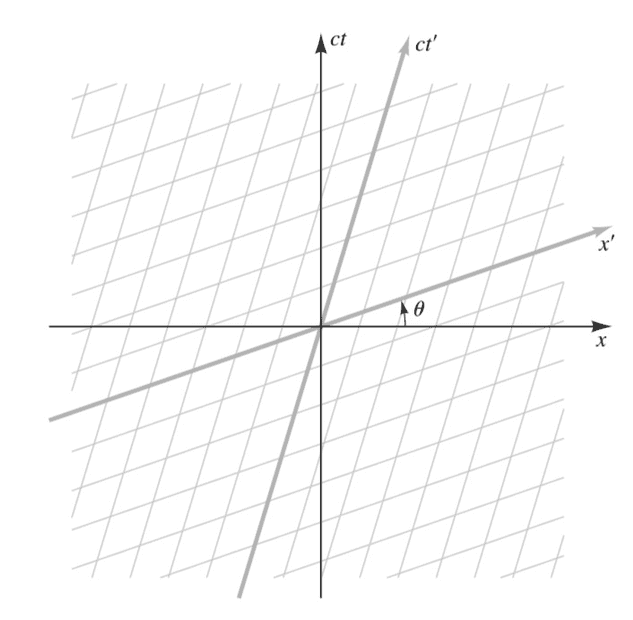(Taking ##ct=y##.)
Equation of ##x'## is given as ##y Cosh\theta=x Sinh\theta ## so
On the line ##x'## we've a point ##y/x=Tanh \theta## ----(1)

This point lies on a hyperbola ##x^2 -y^2=R^2## with ##R^2=x^2(1-Tanh\theta)##

The area of sector is ##
A = \frac{1}{2}x\sqrt{x^2 -R^2} - \int _R ^x \sqrt{x^2 -R^2}dx##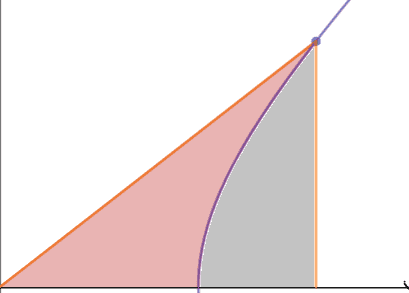##A=\frac{R^2}{2} \ln \left(\frac{x+\sqrt{x^2-R^2}}{R}\right)##

We've defined ##2A/R^2##( Twice area divided by Minkowski length) as the hyperbolic angle ##\beta##, so:

##\beta=\ln \left(\frac{x+y}{R}\right)## -----(2)

For all points on the ##x'## axis we can write ##x=R cosh u, y=R sinh u##-----(3)

we then have from (2) and (3) :
##\beta=u## ----(4)

But also ##y/x = tanh u## and ##y/x=Tanh\theta##( from (1))

So ##β=u=\theta##

##β=\theta##

Hence all points on the line ##x'## have the same hyperbolic angle ##\theta##.

Correct?

And this is supposed to be simpler than teaching (multi-)linear algebra with the Minkowski product as fundamental form of a Minkowski space? For me the deliberation from drawing Minkowski diagrams in high school to tensor algebra and calculus was a revelation.
Yes.
It is developing a variation of the trigonometric techniques that typical introductory students already know.

I think this helps to make relativity accessible to more “students of introductory physics who may not be physics majors”. I’m talking about introducing relativity at the level of Serway (the last few chapters of the intro text or the modern physics text... not Landau.)

It is, I think, a more navigable bridge on the way to the land of "tensorial utopia",
which many of the students I just described will not reach… but that’s okay.
But I think trigonometry will help these students appreciate relativity
better than tensor algebra and tensor calculus would.

Physics majors should at least be exposed to some tensor methods,
but not necessarily at the intro level of Serway.

My target student audience appears to be different from yours.

Last edited:
Just a comment regarding angle as the arc length of the unit circle vs area in the case of the Minkowski geometry:

It is of course possible to reformulate the Euclidean case in terms of the area of the circle sector as well rather than the arc length of the circle segment (the two being related by a factor of two). This makes the approaches even more analogous.
Yes.

"On the definitions of the trigonometric functions" (1894)
Alexander Macfarlane (1851-1913)
https://archive.org/details/ondefinitionstr00macfgoog
tries to develop circular and hyperbolic trigonometry using directed areas.

"On the introduction of the notion of hyperbolic functions" (1895)
Bull. Amer. Math. Soc. 1 (1895), 155-159
https://www.ams.org/journals/bull/1895-01-06/S0002-9904-1895-00266-9/
"If we now apply to the plane the linear transformation
the hyperbola is transformed into itself. The points P and Q are moved to any arbitrary position on the curve, but the ratio ##\alpha_2:\alpha_1## is unaltered. It is easily shown that in this transformation the area of any triangle, and hence the area of any figure in the plane, is unchanged ; so that u, sinh u and cosh u are unchanged. They are therefore all functions of the ratio ##\alpha_2:\alpha_1## alone. Hence sinh u and cosh u are functions of u alone, and the definitions here given are a proper generalization of the usual definitions."
(That's a boost in light-cone coordinates, in the Bondi k-calculus.)

The analogy is due to their projective ancestry,
both Euclidean geometry and Minkowski spacetime geometry are
Cayley-Klein geometries, which unifies the classical non-Euclidean geometries, as well as their Lorentzian signature analogues. https://en.wikipedia.org/wiki/Cayley–Klein_metric
Strategy: use the unified viewpoint, as applied to a familiar geometry,
to learn about a new geometry.

Last edited:
Attempt to find the hyperbolic angle (rapidity) of points on the line ##x'##:
...

Hence all points on the line ##x'## have the same hyperbolic angle ##\theta##.

Correct?
In the spirit of my previous post (mentioning the unified geometrical viewpoint),
repeat your strategy for a circular angle [noting any slight variations that are necessary].
Does it work?

In the spirit of my previous post (mentioning the unified geometrical viewpoint),
repeat your strategy for a circular angle [noting any slight variations that are necessary].
Does it work?
Yes, I'll try that way.
Was my proof using areas ,repeated below correct?
Attempt to find the hyperbolic angle (rapidity) of points on the line ##x'##:
View attachment 314620

(Taking ##ct=y##.)
Equation of ##x'## is given as ##y Cosh\theta=x Sinh\theta ## so
On the line ##x'## we've a point ##y/x=Tanh \theta## ----(1)

This point lies on a hyperbola ##x^2 -y^2=R^2## with ##R^2=x^2(1-Tanh\theta)##

The area of sector is ##
A = \frac{1}{2}x\sqrt{x^2 -R^2} - \int _R ^x \sqrt{x^2 -R^2}dx##

View attachment 314619

##A=\frac{R^2}{2} \ln \left(\frac{x+\sqrt{x^2-R^2}}{R}\right)##

We've defined ##2A/R^2##( Twice area divided by Minkowski length) as the hyperbolic angle ##\beta##, so:

##\beta=\ln \left(\frac{x+y}{R}\right)## -----(2)

For all points on the ##x'## axis we can write ##x=R cosh u, y=R sinh u##-----(3)

we then have from (2) and (3) :
##\beta=u## ----(4)

But also ##y/x = tanh u## and ##y/x=Tanh\theta##( from (1))

So ##β=u=\theta##

##β=\theta##

Hence all points on the line ##x'## have the same hyperbolic angle ##\theta##.

Correct?

correct?

•vanhees71
Yes!

•Kashmir
Just a comment regarding angle as the arc length of the unit circle vs area in the case of the Minkowski geometry:

It is of course possible to reformulate the Euclidean case in terms of the area of the circle sector as well rather than the arc length of the circle segment (the two being related by a factor of two). This makes the approaches even more analogous.
In addition: It is also possible to define the hyperbolic angle as the Lorentzian arc length of the "unit" hyperbola segment.

Source:
https://en.wikipedia.org/wiki/Hyperbolic_angle#Relation_To_The_Minkowski_Line_Element

•vanhees71, Orodruin and robphy
•vanhees71, robphy and Sagittarius A-Star
I'd however stress once more that it's not a usual arclength, and that's why I'd call it "proper time" and only "proper time", which is defined for time-like world lines.

I'd however stress once more that it's not a usual arclength, and that's why I'd call it "proper time" and only "proper time", which is defined for time-like world lines.
You can do the corresponding thing for the spacelike unit hyperbolae and then it is of course not proper time.

•vanhees71
For a spacelike curve you must define it of course with an additional sign under the square root. I can't remember that I've ever needed such a quantity.

For a spacelike curve you must define it of course with an additional sign under the square root. I can't remember that I've ever needed such a quantity.
The minus-sign is needed here if your signature-convention is ##(+---)##.

If the spacelike curve lies in the plane of simultaneity of an inertial observer,
the quantity could be called the "spatial distance" along a spatial path,
a Euclidean arc-length.

A special case of this would be the proper-length of an object at rest with respect to this inertial observer.

•vanhees71
I've just begun learning about space time diagrams.
I'm going all the way back to the original OP. The question was about whether a particular formula generated the value of the ##ct'## coordinate. That question has been answered in spades by all the experts.

There is another approach I came up with to calculate the value of the ##ct'## coordinate, one that doesn't require much math. The experts here may be familiar with it, but I've never seen it anywhere. It relies on Einstein's first postulate: The laws of physics have the same form in all inertial reference frames.

Here is the basic problem. We have an event whose rest coordinates are known; this implies we know the units of the ##x## and ##ct## axes. We want to know the event's ##ct'## value.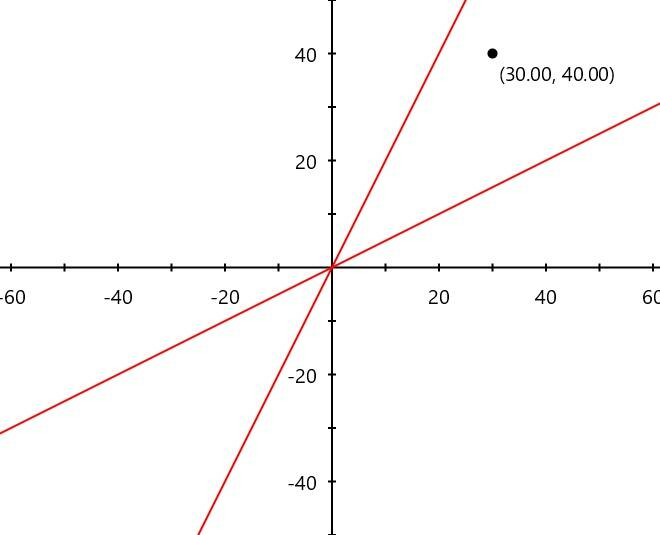I've drawn the ##x'## and ##ct'## axes in red, but not added labeled tick marks. We don't even know the relative velocity of the moving observer (although it could be calculated from the angle of the axes).

The first step is to draw a line parallel to the ##x'## axis through the event. All points on this line have the same ##ct'## value (the same time value as calculated by the moving observer). We don't know this value, but we do know the value where the line crosses the ##ct## axis.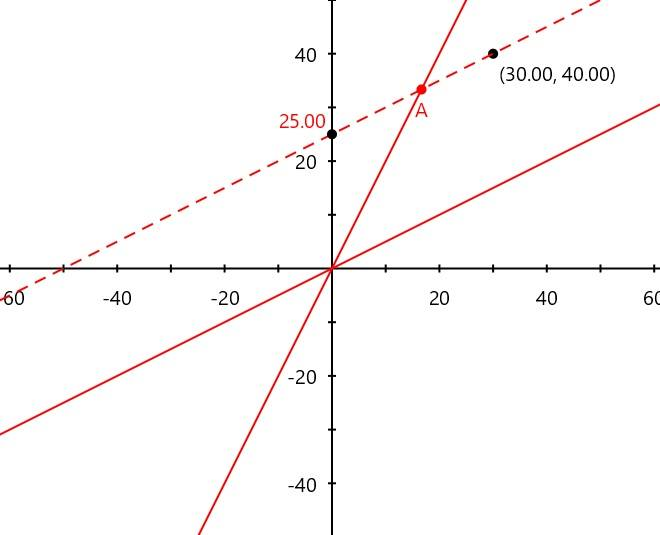We want to know the length from the origin to A (as has been pointed out, this is not a Euclidean length). Draw another line parallel to the ##x## axis. Again, we know where this line crosses the ##ct## axis. All points on this line have this same time value as calculated by the rest observer.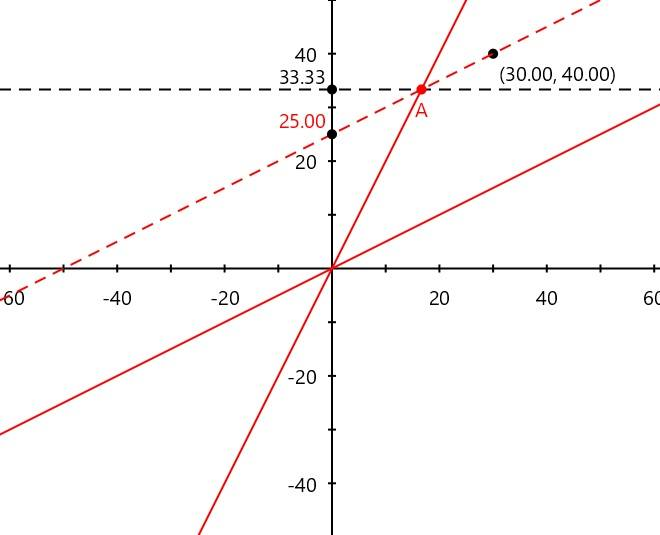Using Einstein's first postulate, we know that the ratio 33.33:A must be the same as the ratio of A:25. Each represents how each observer views the other's clock rate. Therefore, we just need to solve for A in the equation ##33.33/A = A/25##. The answer is ##A = \sqrt{33.33/25} = 28.87## (approximately).

At one point, I wanted to see if I could explain how to generate a basic Minkowski spacetime diagram (essentially, starting with a rest observer and an observer moving at some given relative velocity, draw the axes, their tick marks, and the worldlines of the two observers) starting from Einstein's S.R. postulates, and without requiring any math. Labeling the units for the axes of the moving observer eluded me for some time; the method shown here fails to avoid all math--I still need a division and a square root--but it's as simple as I could get.

•Dale and vanhees71
Labeling the units for the axes of the moving observer eluded me for some time; the method shown here fails to avoid all math--I still need a division and a square root--but it's as simple as I could get.
The way to think about this is to ask yourself how you would do it in Euclidean space with a straightedge and a compass (no graded ruler, which effectively doubles as both).

Hint: You essentially need to find a way to draw hyperbolae.

•Nugatory and vanhees71
The way to think about this is to ask yourself how you would do it in Euclidean space with a straightedge and a compass (no graded ruler, which effectively doubles as both).

Intriguing. But before I go off to drawing hyperbolas by geometric construction, I would have to figure out how to relate that to Einstein's postulates. That there is a connection is certain; that the connection is straight-forward is not. It is not enough to come up with a method of finding the tick marks on the moving observer's axes--the reason the method works has to be given, and the explanation can't involve a complex mathematical derivation.

In my system, the explanation directly ties to Einstein's postulate; labeling the point on the ##ct'## axis requires some simple math (you need to know about ratios, square roots, and division). If you have a method that avoids the math altogether, that would be great.

Einstein's postulates can for sure be fulfilled by simply assuming that the quadratic form on four-vectors, ##\eta_{\mu \nu} x^{\mu} x^{\nu}## with ##(\eta_{\mu \nu}=\mathrm{diag}(1,-1,-1,-1)## is invariant under boosts (i.e., transforming from one inertial frame of reference to another). That's so, because then the front of a spherical em. wave (light signal) obeys ##c^2 t^2-\vec{x}^2=0## in all inertial frames of reference, and indeed with this assumption you can construct Minkowski space with the Lorentz transformations as the symmetry group (or more precisely the Poincare group, because this space is also homogeneous, i.e., translation invariant).

This implies that the temporal/spatial units on the axes of inertial frames depicted in your Minkowski diagram are determined by the corresponding unit hyperbolae ##c^2 t^2-x^2=\pm 1##, i.e., the intersection of these hyperbolae determine the unit-tic marks on the time and space axes of all inertial reference frames.

These unit hyperbolae are analogous to the unit circle, with which you construct the units on the axes of Cartesian coordinate systems in the Euclidean plane, and the symmetry in this plane are rotations rather than Lorentz transformations of course.

•Dale
Einstein's postulates can for sure be fulfilled by simply assuming that the quadratic form on four-vectors

My response to @Kashmir was triggered by the fact that he was starting to learn about spacetime diagrams. I offered my solution to finding the ##ct'## coordinate because I think it's an interesting alternative that applies Einstein's postulate directly.

I do have a personal challenge to explain the basic elements of Minkowsky diagrams to someone with only basic math knowledge (mentioning the "quadratic form of four vectors" would exceed the limits I had in mind) . This is just for my entertainment, and pursuing how one might do that (and what level of knowledge would be allowed) seems like hijacking @Kashmir's thread. If I decide it's worth discussing, I will post my own thread.

•vanhees71
But before I go off to drawing hyperbolas by geometric construction, I would have to figure out how to relate that to Einstein's postulates.
It comes almost directly from the 2nd postulate. Start with the spacetime interval: $$\Delta s^2 = -c^2 \Delta t^2 +\Delta x^2 + \Delta y^2 + \Delta z^2$$ Note that this is the equation for a hyperboloid. If ##\Delta s^2 > 0 ## it is a hyperboloid of one sheet, and if ##\Delta s^2 <0 ## it is a hyperboloid of two sheets. Now, for the special case of ##\Delta s^2 = 0## we have a degenerate hyperboloid, a cone. Up to this point this is just geometry. If we rearrange the special case cone then we can get: $$c^2 \Delta t^2 = \Delta x^2 + \Delta y^2 + \Delta z^2$$ which we recognize as a sphere with radius ##c \Delta t##. In other words, this is the equation of a flash of light (which we call a light cone). The light expands outward in a sphere at a speed of ##c##. The first postulate is embodied in the fact that ##\Delta s^2## is invariant, meaning that if something travels at the speed of light in any direction in one reference frame then it travels at the speed of light in all reference frames.

•Freixas and vanhees71
Hint: You essentially need to find a way to draw hyperbolae.
That's what the light-clock diamonds do... they are proxies for hyperbolas.

Constructed using the light-signals in a light-clock,
and motivated by the Bondi k-calculus (using the speed of light postulate and the relativity-postulate).
Their edges are lorentz-invariant directions (in (1+1)-Minkowski spacetime), since they are along eigenvectors of a boost. Since the determinant is one, [since the eigenvalues (stretch factors for the eigenvectors) are reciprocals] the area of the diamond is lorentz-invariant.

Here's my least technical introduction
https://www.physicsforums.com/insights/relativity-on-rotated-graph-paper-a-graphical-motivation/

•Freixas, Dale and vanhees71
They are not proxies but exact. In light-cone coordinates the hyperbolae are parametrized as
$$x^+=\frac{A}{x^-},$$
with ##A## a constant area.

In light-cone coordinates the Lorentz boost reads
$$x^{\prime +}=\kappa x^{+}, \quad x^{\prime -}=\frac{1}{\kappa} x^{-}, \quad \kappa=\exp(-\eta),$$
where ##\eta## is the rapidity. In the usual Lorentzian components it reads
$$x^{\prime 0}=\cosh \eta x^0 - \sinh \eta x^1, \quad x^{\prime 1} =-\sinh \eta x^0 + \cosh \eta x^1.$$
That simple algebra is what's behind your "rotated-graph paper" geometric constructions.

•Freixas
Thank you all […]
You asked for help. Now, in turn, you can help this Forum. Honestly, how much did this thread manage to illuminate the topic you started?

You asked for help. Now, in turn, you can help this Forum. Honestly, how much did this thread manage to illuminate the topic you started?
It helped clear that the diagram was to be interpreted not read off directly. And the angles were not circular but hyperbolic and how we calculate it.

Then the discussion went on between those participants which was beyond me.

•robphy, apostolosdt, vanhees71 and 1 other person